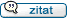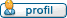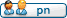Das deutsche QBasic- und FreeBASIC-Forum
Für euch erreichbar unter qb-forum.de, fb-forum.de und freebasic-forum.de!

 Zur Begleitseite des Forums / Chat / Impressum Aktueller Forenpartner:

Autor Nachricht
bauigel

Anmeldungsdatum: 09.02.2018
Beiträge: 7
Wohnort: 75196 RemchingenVerfasst am: 11.09.2023, 18:29    Titel: Label in Zeile 570 not definedHallo Programmierer, was verursacht diesen Fehl in meinem Programm: 10: Rem DLT DURCHLAUFTRAEGER 20: Read N 25: LPrint "N="; N 30: Dim N(N), L(N), WO(N), F1(N), K(N), A(N), B(N), CW(N), C(N), FL(N), FR(N), FA(N), U(4, 4) 40: For J = 1 To N 50: Read N(J) 55: LPrint "N"; J; "="; N(J) 60: N(J) = N(J - 1) + N(J) 70: Next J 75: LPrint B0: NG = N(N) 90: Dim DX(NG), QL(NG), QR(NG), F(NG), X(NG), Q(NG), FQ(NG), M(NG), P(NG), W(NG) 100: For I = 1 To NG 110: Read DX(I), QL(I), QR(I), F(l) 112: LPrint "DX="; DX(I) 113: LPrint "QL="; QL(I) 114: LPrint "QR="; QR(I) 115: LPrint "F= "; F(I) 116: LPrint 120: X(I) = X(I - 1) + DX(I) 130: QL(I) = (QR(I) - QL(1)) / DX(J) 140: Next I 150: For J = 1 To N 160: L(J) = X(N(J)) - X(N(J - 1)) 170: FL(J) = F(N(J)) 180: Next J 190: Input "ELASTISCH GESTUETZT: J/N? "; E\$ 191: If E\$ <> "J" And E\$ <> "N" Then 190 200: If E\$ = "N" Then 260 210: For J = 0 To N 220: Read CW(J) 225: LPrint "CW"; J; "="; CW(J) 230: Next J 240: Read DL, DR 242: LPrint "DL="; DL 243: LPrint "DR= "; DR 245: LPrint 250: L\$ = "L": R\$ = "R": GoTo 280 260: Input "LINKS EINGESPANNT:J /N ? "; L\$ 261: If L\$ <> "J " And L\$ <> "N" Then 260 270: Input "RECHTS EINGESPANNT: J/N?"; R\$ 271: If R\$ <> "J" And R\$ <> "N" Then 270 280: If L\$ = "J" Or DL >= 1E20 Then 300 290: Input "MOMENT LINKS: "; ML 295: LPrint "ML="; ML 300: If R\$ = "J" Or DR >= 1E20 Then 320 310: Input "MOMENT RECHTS : "; MR 315: LPrint "MR="; MR 320: If E\$ = "J " Then 400 330: Input "STUETZENSENKUNG: J/N?"; S\$ 331: If S\$ <> "J" And S\$ <> "N" Then 330 340: If S\$ = "N" Then 400 350: Sleep 1 360: Input "J="; J 370: Print "WO"; J; "" 380: Input WO(J): 385: LPrint "WO"; J; "="; WO(J) 390: GoTo 330 400: Input "BIEGESTEIFIGKEIT E*IO="; B 404: LPrint 405: LPrint "E*IO="; B 410: Input "KONST. QUERSCHNITT: J/ N? "; Q\$ 411: If Q\$ <> "J" And Q\$ <> "N" Then 410 420: Sleep 1: C(0) = CW(0) / B 430: DO1 = DL / B: DN = DR / B 440: For J = 1 To N 450: If Q\$ = "J" Then Let K(J) = 1: GoTo 480 460: Print "I"; J; "/I0='';" 470: Input K(J): Print 475: LPrint "J/JO="; K(J) 480: If E\$ = "N" Then 500 490: C(J) = CW(J) / B 500: F(N(J)) = F1(J) 510: Next J 515: LPrint 520: For J = 1 To N 530: FQ(N(J - 1)) = 0: M(N(J - 1)) = 0 540: P(N(J - 1)) = 0: W(N(J - 1)) = 0 550: For I = 1 + N(J - 1) To N(J) 560: T = DX(I) 570: GoSub ZG 580: FQ(I) = FQ - F(I): M(I) = M 590: P(I) = P: W(I) = W 600: Next I 610: If E\$ = "J" Then 910 615: Rem STARRE LAGERUNG 620: C(J) = 6 * K(J) / L(J) 630: DW = (W(N(J)) - B * (WO(J) - WO(J - 1))) / L(J) 640: A(J) = M(N(J)) + C(J) * DW 650: B(J) = P(N(J)) - 3 * DW 660: FR(J - 1) = C(J) * DW / L(J) 670: FL(J) = FQ(N(J)) 680: Next J 690: For U = 1 To 3 700: If U = 1 Then 820 710: GoSub SL 720: If U = 3 Then 890 730: If R\$ = "J" Then 760 740: C = (MR - M(N)) / M1 750: GoTo 770 760: C = -P(N) / P1 770: If L\$ = "J" Then 800 780: M(0) = ML: P(0) = C 790: GoTo 890 800: M(0) = C: P(0) = 0 810: GoTo 890 820: If L\$ = "J" Then 850 830: M(0) = 0: P(0) = 1 840: GoTo 860 850: M(0) = 1: P(0) = 0 860: GoSub SL 870: M1 = M(N): P1 = P(N) 880: M(0) = ML: P(0) = 0 890: Next U 900: GoTo 1480 910: Rem ELASTISCHE STUETZEN 920: FL(J) = FQ(N(J)) 930: A(J) = M(N(J)) 940: B(J) = P(N(J)) 950: WO(J) = W(N(J)) 960: J = J + 1 970: For S = 0 To 4 980: For J = 1 To N 990: FQ(J) = FQ(J - 1) + C(J - 1) * W(J - 1) 1000: M(J) = L(J) * FQ(J - 1) + M(J - 1) + C(J - 1) * L(J) * W(J - 1) 1010: P(J) = P(J - 1) - (2 * M(J - 1) + L(J) * (FQ(J - 1) + C(J - 1) * W(J - 1))) * L(J) / 2 / K(J) 1020: W(J) = P(J - 1) - (M(J - 1) + FQ(J - 1) * L(J) / 3) * L(J) / 2 / K(J) * L(J) 1030: W(J) = W(J) + (1 - L(J) ^ 3 / 6 / K(J) * C(J - 1)) * W(J - 1) 1040: If S <> 0 Then 1090 1050: FQ(J) = FQ(J) + FL(J) 1060: M(J) = M(J) + A(J) 1070: P(J) = P(J) + B(J) 1080: W(J) = W(J) + WO(J) 1090: Next J 1100: V(1, S) = FQ(N) 1110: V(2, S) = M(N) 1120: V(3, S) = P(N) 1130: V(4, S) = W(N) 1140: If S = 4 Then 1220 1150: If S = 3 Then 1210 1160: If S = 2 Then 1200 1170: If S = 1 Then 1190 1180: FQ(0) = 1: GoTo 1220 1190: FQ(0) = 0: M(0) = 1: GoTo 1220 1200: M(0) = 0: P(0) = 1: GoTo 1220 1210: P(0) = 0: W(0) = 1 1220: Next S 1230: A1 = V(1, 3) - DO1 * V(1, 2) + C(N) * V(4, 3) - DO1 * V(4, 2) 1240: B1 = V(1, 4) + C(N) * V(4, 4) 1250: C1 = V(1, 0) + V(1, 2) * ML + C(N) * V(4, 0) + V(4, 2) * M(1) 1260: A2 = V(2, 3) - DO1 * V(2, 2) - DN * (V(3, 3) - DO1 * V(3, 2)) 1270: B2 = V(2, 4) - DN * V(3, 4) 1280: C2 = V(2, 0) + V(2, 2) * ML - DN * (V(3, 0) + V(3, 2) * ML) - MR 1290: DET = A1 * B2 - A2 * B1 1300: P(0) = (C2 * B1 - C1 * B2) / DET 1310: W(0) = (A2 * C1 - A1 * C2) / DET 1320: FQ(0) = C(0) * W(0): FA(0) = FQ(0) 1330: M(0) = ML - DD * P(0) 1340: If CW(0) = 1E20 Then Let W(0) = 0 1350: If DL >= 1E20 Then Let P(0) = 0 1360: For J = 1 To N 1370: For I = 1 + N(J - 1) To NU(J) 1380: T = DX(I) 1390: GoSub ZG 1400: FQ(I) = FQ - F(I): M(I) = M 1410: P(I) = P: W(J) = W 1420: Next I 1430: FA(J) = C(J) * W(N(J)) 1440: FA(N) = -(FQ(NG)) 1450: FQ(N(J)) = FQ(N(J)) + FA(J) 1460: F(N(J)) = F1(J) - FA(J) 1470: Next J 1480: LPrint "AUFLAGERKRAEFTE:" 1490: For J = 0 To N 1500: If E\$ = "N" Then Let FA(J) = FR(J) - FL(J) 1510: LPrint "FA"; J; "="; FA(J) 1520: F(N(J)) = F1(J) - FA(J) 1530: Next J 1535: LPrint 1540: FQ(0) = FA(0) 1550: I = 0: 1555 GoSub AUSGABE 1560: MM = ABSM(0): XM = 0 1570: For J = 1 To N 1580: For I = 1 + N(J - 1) To N(J) 1590: T = DX(I) 1600: GoSub ZG 1610: FQ(I) = FQ - F(I): M(I) = M5 1620: P(I) = P: W(I) = W 1630: If MM > Abs(M5) Then 1650 1640: MM = Abs(M5): XM = X(I) 1650: If Q1(I) = 0 Then 1700 1660: R = 2 * Q1(I) * FQ(I - 1) + QL(I) * QL(I) 1670: If R < 0 Then 1790 1680: T = (Sqr(R - QL(I))) / Q1(I) 1690: GoTo 1720 1700: If QL(I) = 0 Then 1790 1710: T = FQ(I - 1) / QL(I) 1720: If T < 0 Or T > DX(I) Then 1790 1730: Rem GoSub ZG 1740: LPrint "X="; X(I - 1) + T 1750: LPrint "M="; M 1755: LPrint 1760: If MM > ABSM(5) Then 1790 1770: MM = Abs(M) 1780: XM = X(I - 1) + T 1790: GoSub AUSGABE 1800: Next I 1810: Next J 1820: LPrint "MAX M="; MM 1830: LPrint "BEI X="; XM 1835: LPrint 1840: Input "NEUE QUERSCHNITTE: J/N? "; NQ\$ 1841: If NQ\$ <> "J" And NQ\$ <> "N" Then 1840 1850: If NQ\$ = "J" Then 400 1860: Input "VERFORMUNG: J/N?"; U\$ 1861: If U\$ <> "J" And U\$ <> "N " Then 1860 1870: If U\$ = "N" Then 2080 1880: Input "AEQUID.STELLEN: J/N? "; A\$ 1881: If A\$ <> "J" And A\$ <> "N" Then 1880 1890: If A\$ = "J" Then 1920 1900: Input "X="; X 1910: N0 = 0: GoTo 1940 1920: Input "ANZAHL DER INTERVALLE? "; NO 1930: DX = X(NG) / NQ: X = 0 1940: For J = 1 To N 1950: For I = 1 + N(J - 1) To N(J) 1960: If X > X(I) Then 2040 1970: T = X - X(J - 1) 1980: GoSub VG 1990: LPrint "X="; X 2000: LPrint "PHI= "; P / 8 2010: LPrint "W='';W/8" 2015: LPrint 2020: If N0 = 0 Then 2060 2030: X = X + DX: GoTo 1960 2040: Next I 2050: Next J 2060: Input "WEITERE VERFORMUNG :J/N?"; WU\$ 2061: If WU\$ <> "J " And WU\$ <> "N" Then 2060 2070: If WU\$ = "J " Then 1880 2080: End Sub ZG 2270: FQ = FQ(I - 1) - (QL(I) + Q1(I) * T / 2) * T 2280: M = M(I - 1) + (FQ(I - 1) - (QL(I) + Q1(I) * T / 3) * T / 2) * T 2285: Return End Sub Sub AUSGABE 2200: Rem "AUSGABE": LPRINT "X="; X(I) 2210: If I = 0 Then 2240 2220: LPrint "FQL="; FQ(I) + F(I) 2230: If I = NG Then 2250 2240: LPrint "FQR= "; FQ(I) 2250: LPrint "M="; M(I) 2255: LPrint 2260: Return End Sub Sub VG 2290: P = P(I - 1) - (M(I - 1) + (FQ(I - 1) - (QL(I) + Q1(I) * T / 4) * T / 3) * T / 2) * T / K(J) 2300: W = W(I - 1) + (P(I - 1) - (M(I - 1) + (FQ(I - 1) - (QL(I) + Q1(I) * T / 5) * T / 4) * T / 3) * T / 2 / K(J)) * T 2310: Return End Sub Sub SL 2320: For J = 1 To N 2330: M(J) = -2 * M(J - 1) + C(J) * P(J - 1) 2340: P(J) = 3 / C(J) * M(J - 1) - 2 * P(J - 1) 2350: If U = 1 Then 2410 2360: M(J) = M(J) + A(J) 2370: P(J) = P(J) + B(J) 2380: If U <> 3 Then 2410 2390: FR(J - 1) = FR(J - 1) + (C(J) * P(J - 1) - 3 * M(J - 1)) / L(J) 2400: FL(J) = FR(J - 1) + FL(J) 2410: Next J 2420: Return End SubHorstD

Anmeldungsdatum: 01.11.2007
Beiträge: 102Verfasst am: 12.09.2023, 09:32    Titel:Eine Sub wird nicht mit GOSUB aufgerufenBeiträge der letzten Zeit anzeigen: Alle Beiträge1 Tag7 Tage2 Wochen1 Monat3 Monate6 Monate1 Jahr Die ältesten zuerstDie neusten zuerst
 Alle Zeiten sind GMT + 1 Stunde Seite 1 von 1

 Gehe zu: Forum auswählen Intern----------------Ankündigungen und Neuigkeiten QBasic/QuickBASIC----------------Allgemeine Fragen zu QBasic.Spezielle Fragen FreeBASIC----------------Allgemeine Fragen zu FreeBASIC.Windows-spezifische FragenLinux-spezifische FragenBibliotheken QB und FB für Profis----------------Profi-Forum Verschiedenes----------------ProjektvorstellungenComputer-ForumOff-Topic-Forum
Du kannst keine Beiträge in dieses Forum schreiben.
Du kannst auf Beiträge in diesem Forum nicht antworten.
Du kannst deine Beiträge in diesem Forum nicht bearbeiten.
Du kannst deine Beiträge in diesem Forum nicht löschen.
Du kannst an Umfragen in diesem Forum nicht mitmachen.